# How to adjust the solubility of one oral drug according to cyclodextrin concentration #482

Open
opened this issue Mar 18, 2020 · 10 comments
Open

# How to adjust the solubility of one oral drug according to cyclodextrin concentration#482

opened this issue Mar 18, 2020 · 10 comments
Labels

###wangwei1619 commented Mar 18, 2020

 Hi, all The cyclodxtrin (CD) is an often used solubilization method and can enhance drug solubility depending on CD concentration. As the CD transfers along GI tract, the concentration of CD in GI segments are different from each other and varing with time, and result in change of drug solubility in GI tract. So, is there anyway to consider this impact on solubility in MoBi? I have made a simulation where drug and CD are taken orally together. The drug solubitlity in duodenum is defined by a formula: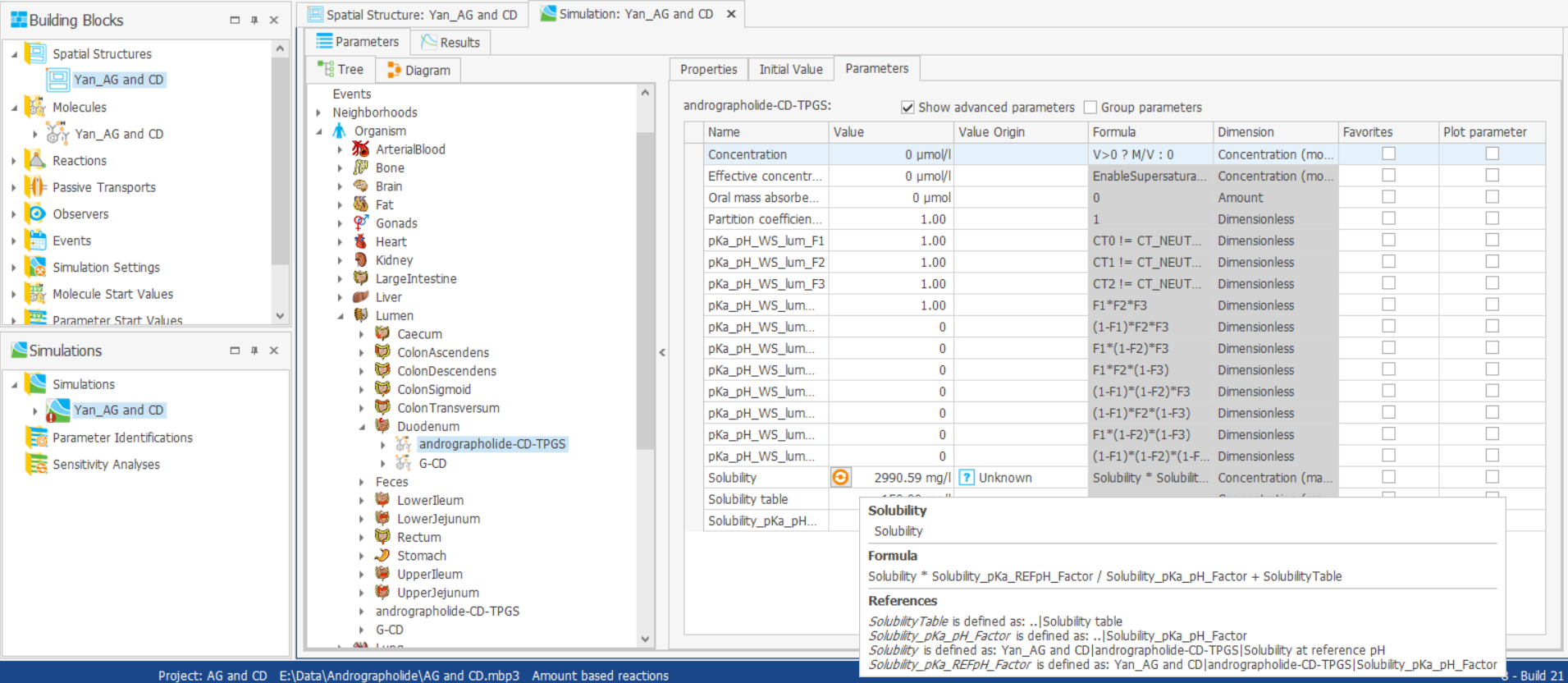And this fomula is also can be seen in spatial structure: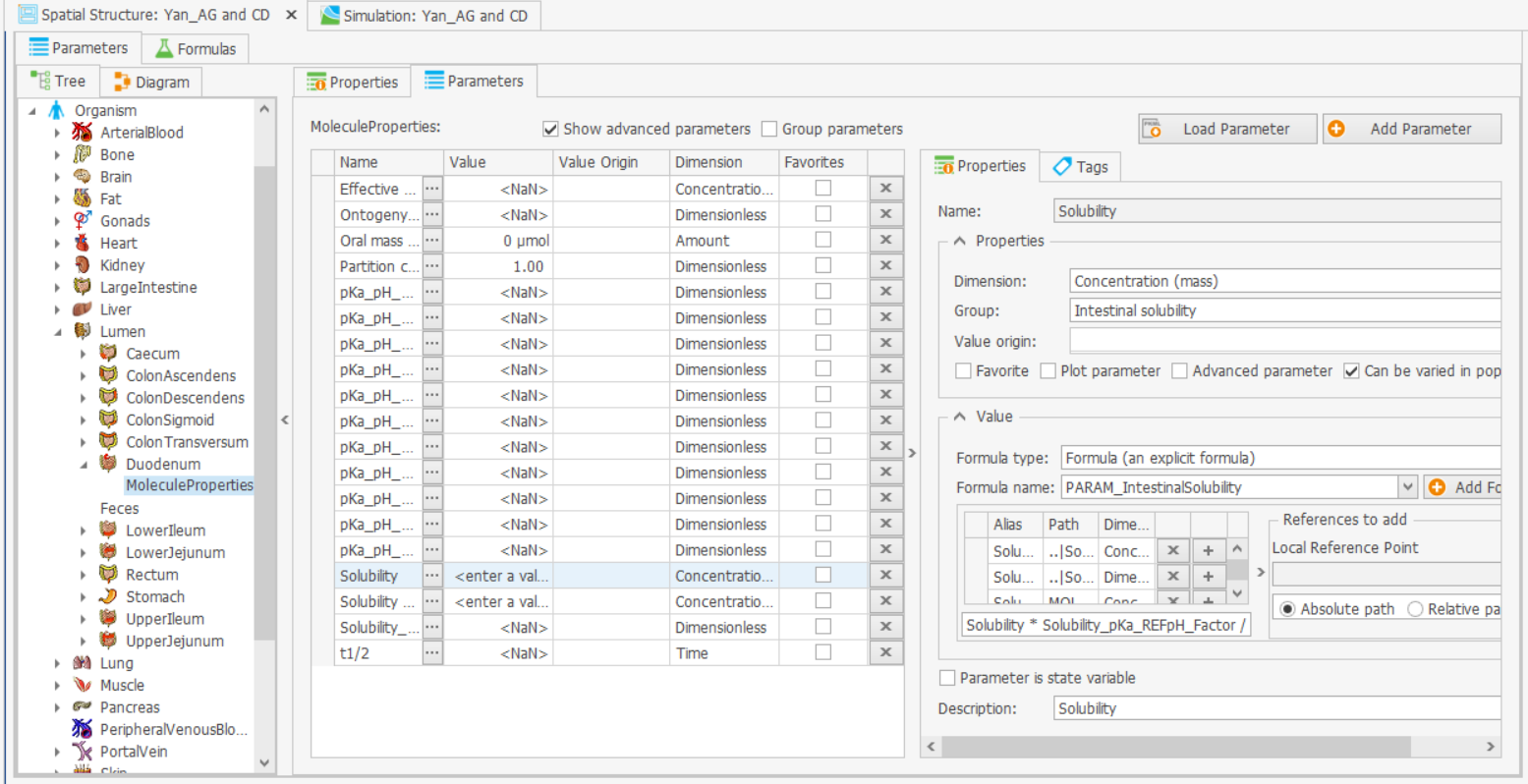I think this formula does the same thing to both drug and CD and is what expected for CD solubility. So, how to adjust the solubility of drug according to CD concentration? The formulas in simulation block seems not could be changed. Thanks for comment.

###jzq90 commented Mar 25, 2020

 How about using the concentration of CD in the GIT to modify the solubility of the drug? Also, I think the influence of CD on the absorption is much more complicated. For one thing, it increases the dissolution rate rather than increases the solubility. For another, the drug entrapped in CD is not free, which can not penetrate the intestine membrane.

###wangwei1619 commented Mar 25, 2020

 That is really ture. Anyway, I think it requires the modeifacation to default built-in equation to consider the impact of CD

###PavelBal commented Mar 25, 2020

 Hi, an interesting setting. First of all, you cannot change the equation in the simulation itself, you have to do it in the Spatial Structure Building Block (BB) and then update your simulation. As you can see, in the Spatial Structure BB, the parameter `Solubility` is located under the entry `MoleculeProperties`. This means that this parameter will be created for each molecule in the simulation, using the same formula. So, if you change the formula, it will be changed for each orally administered molecule. You could create a parameter in the molecule that turns the effect of CD on or off, and further parameters you need to describe the effect. E.g., assuming a Michaelis-Menten-like effect, you would define per molecule the parameters: CD_effect_active (values 0 or 1) CD_effect_Km CD_effect_Vmax Then in the formula you could use something like: `(CD_effect_active = 0 ? 1 : Vmax * C_cd / (C_cd / Km)) * Solubility * Solubility_pKa_REFpH_Factor / Solubility_pKa_pH_Factor + SolubilityTable` To generically access the parameter value of the molecule for which the formula is calculated, use the key-word `MOLECULE` in the path-definition of the aliases (variables) of the equation, while `C_cd` should always be the concentration of CD: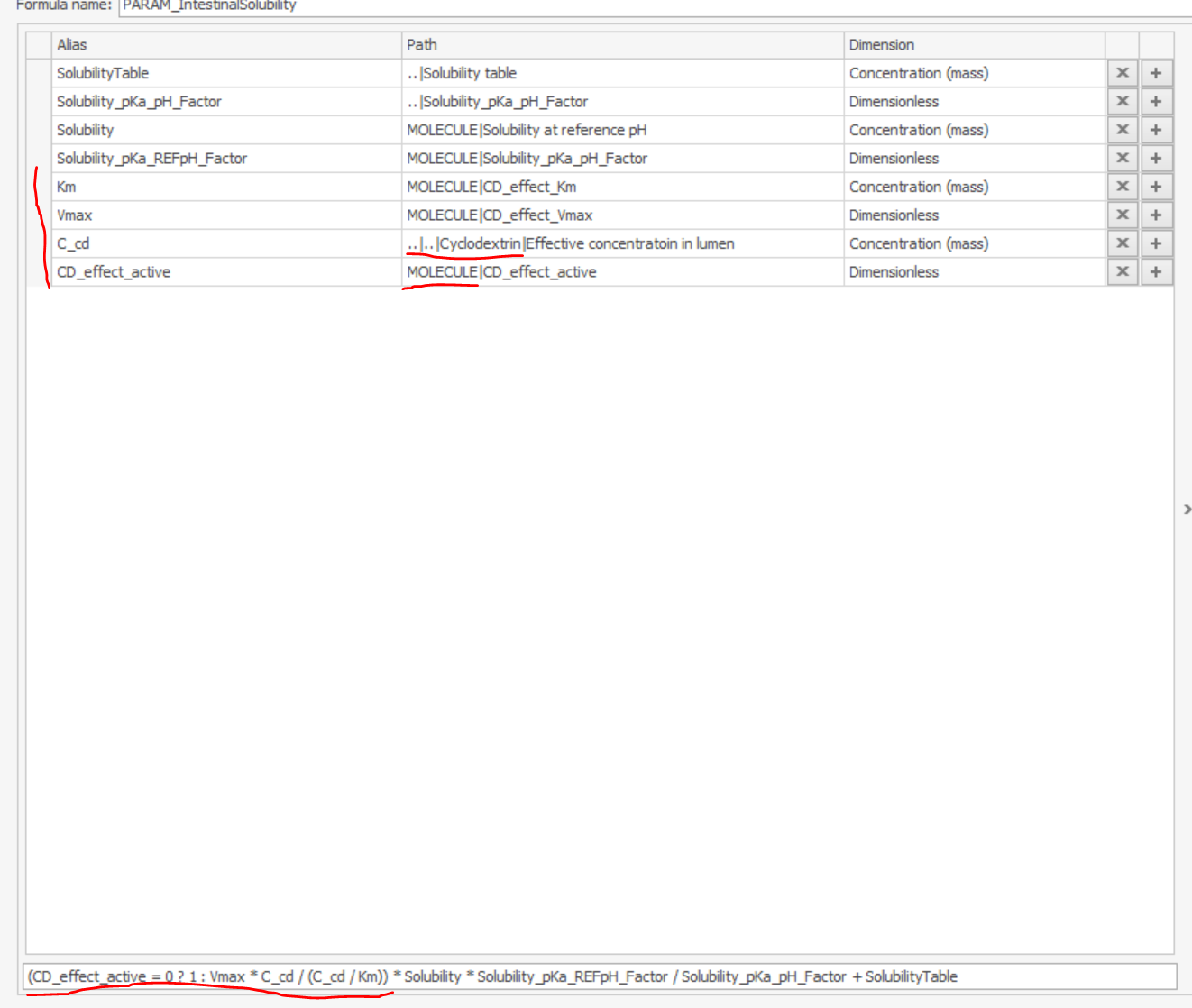So, for CD itself, you would set the value of `CD_effect_active` to zero, for the affected molecule to one. Let me know if it works!

###PavelBal commented Mar 25, 2020

 The dimensions of Km and C_cd should be `Concentration (molar)`, and not `Concentration (mass)`, as in the screenshot.

###wangwei1619 commented Mar 26, 2020

 Wow!! Genius!! @PavelBal , Thanks for your suggestion, I will try it later. So, is what you mean first to creat a parameter in Molecule BB to switch the effect of CD on or off, then modify the solubility equation in Spatial Strucure BB ?

###PavelBal commented Mar 26, 2020

 Just experience ;) Yes, exactly - create the parameters describing the effect of CD in the Molecule BB, and then adjust the formula in the spatial structure BB.

###msevestre commented Mar 26, 2020

 Wow!! Genius!! @PavelBal , agreed.

###wangwei1619 commented Mar 30, 2020

 Oh, I meet a few bit problems when I try this method. I want to use this equation to calculate solubility: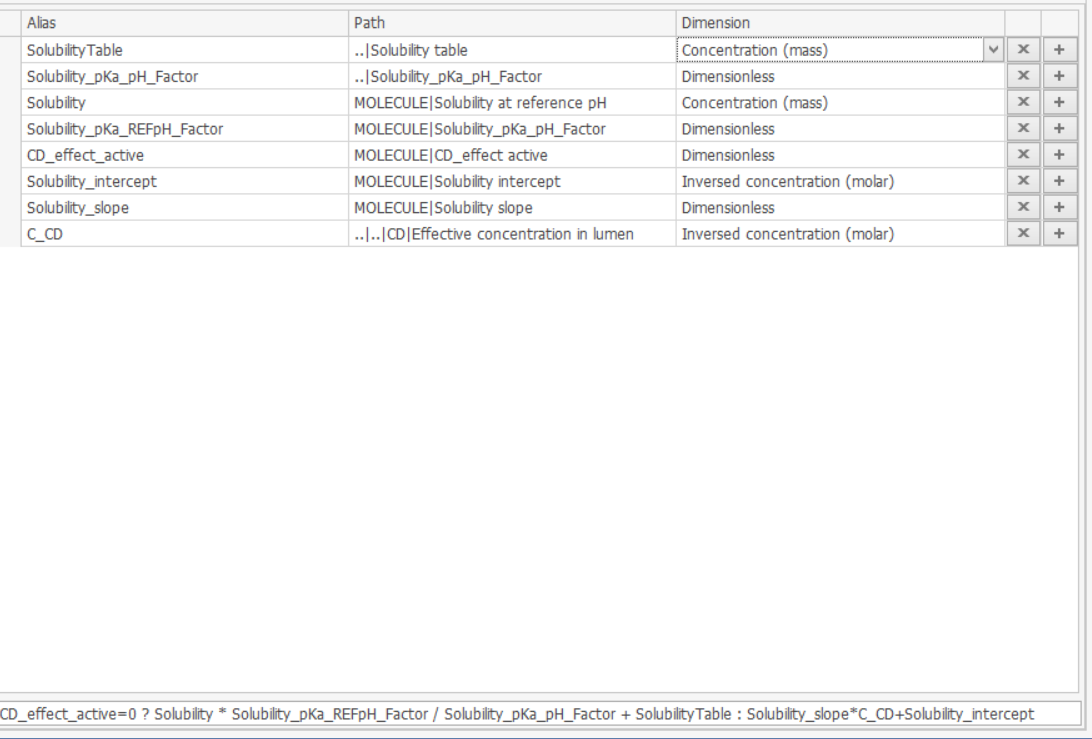The parameters used to CD molecule are with dimension of concentration (mass) as default, but parameters for drug molecule are with dimension of concentration (molar) thus the calculated solubility should be with molar dimension. Would this make a mistake for program since the solubility is in mass dimension by default?

###wangwei1619 commented Mar 30, 2020

 And the second question is if I have a parameter defined in Molecule BB in dimension of concentration (mass) , can I refer to it as dimension of concentration (molar) in formulas elsewhere?

###wangwei1619 commented Mar 30, 2020

 Opps! When I configure the simulation using modified BB, I get this note: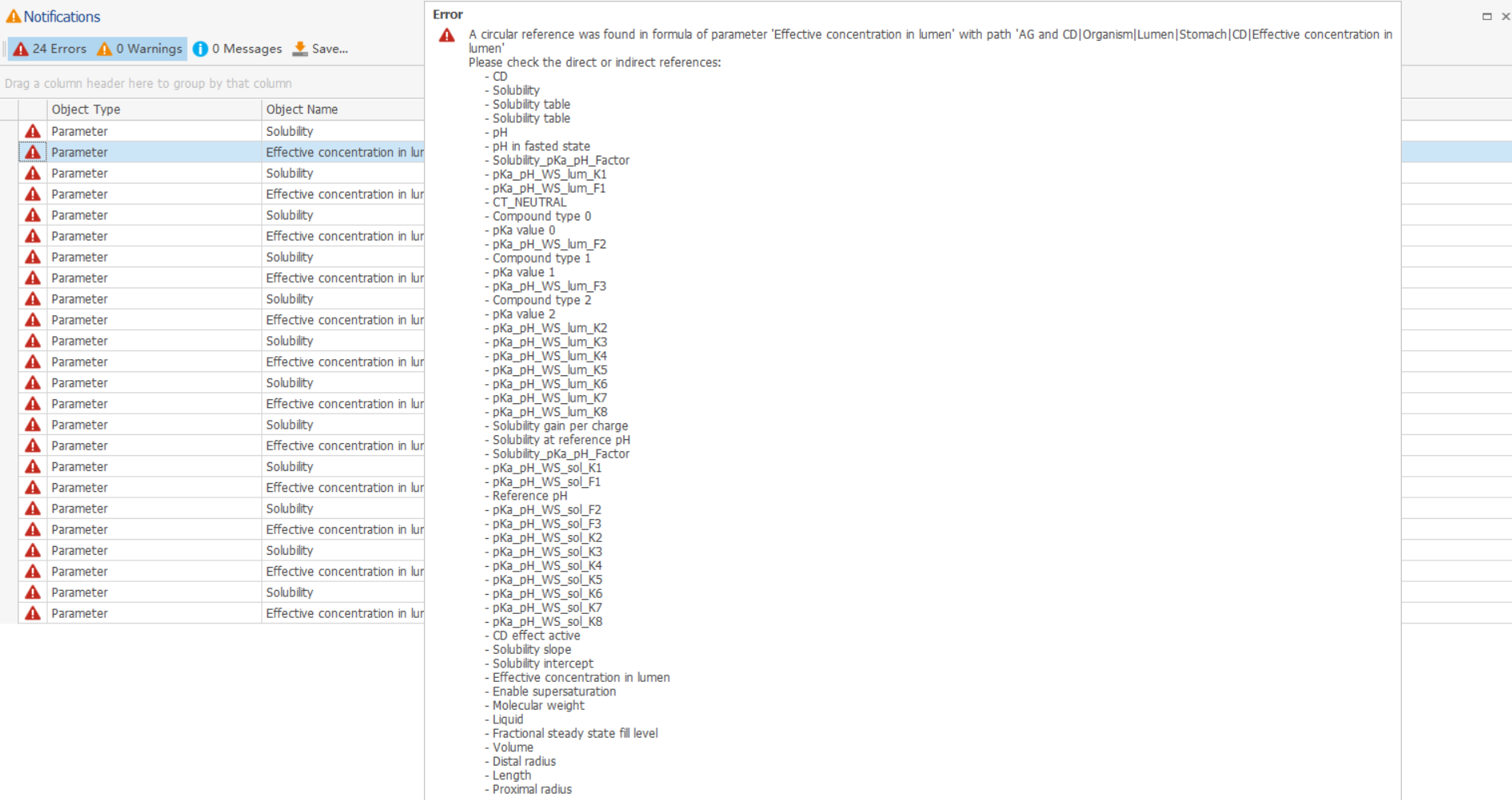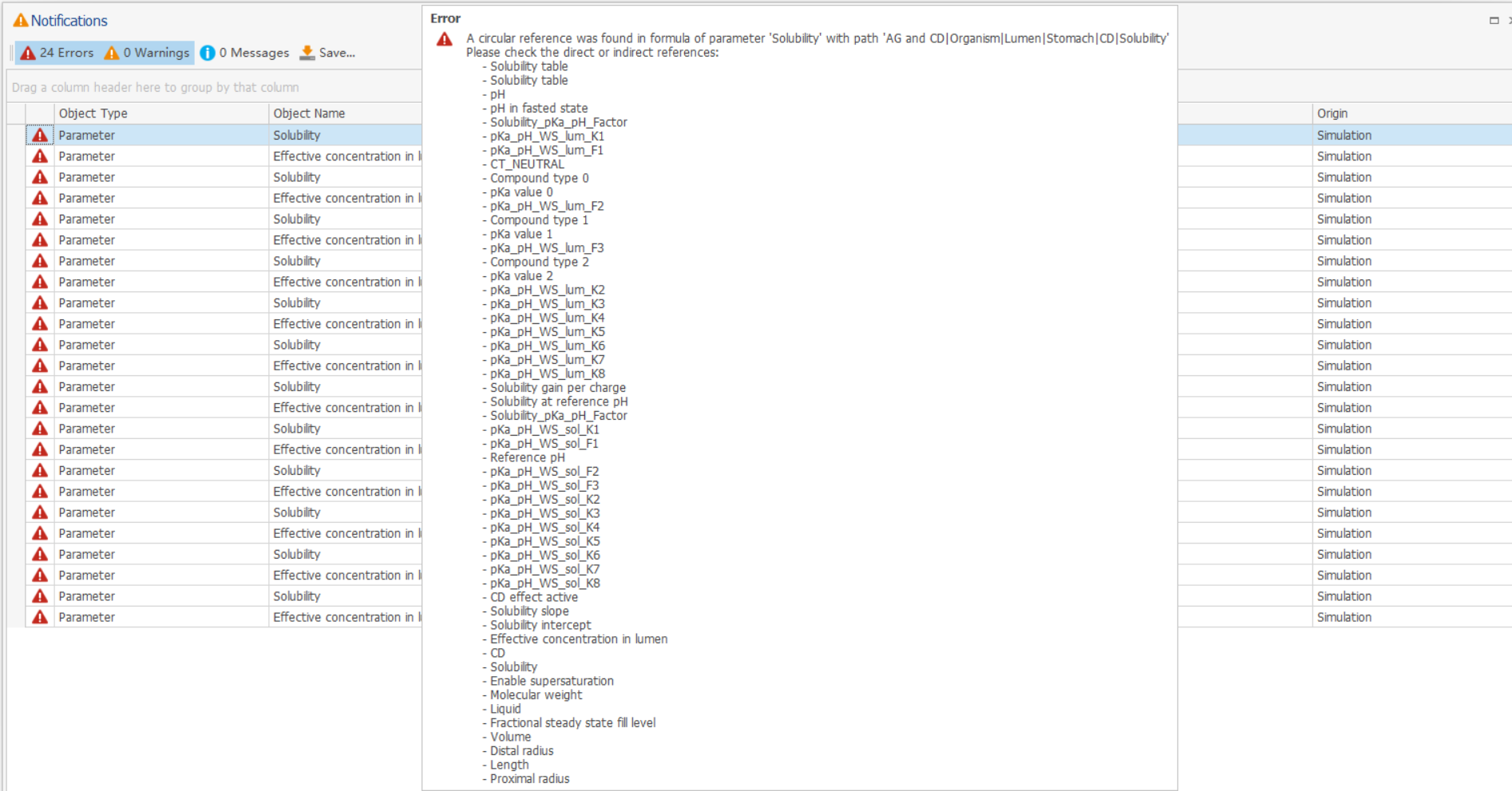This would happen after I add new alias into formula "PARAM_IntestinalSolubility" and ignores whether the original equation is changed or not. Does MoBi scans parameters prior to loading the equation and conclude I am circularly refering parameters? Can I avoid this? And is this problem related to the Parameter type and Group I chose when I created parameters in Molecule BB?